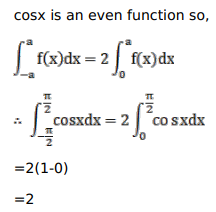# Mark against the correct answer in the following:Question:

Mark $(\sqrt{)}$ against the correct answer in the following:

$\int_{-\pi / 2}^{\pi / 2} \cos x d x=?$

A. 0

B. 2

C. -1

D. none of these

Solution: